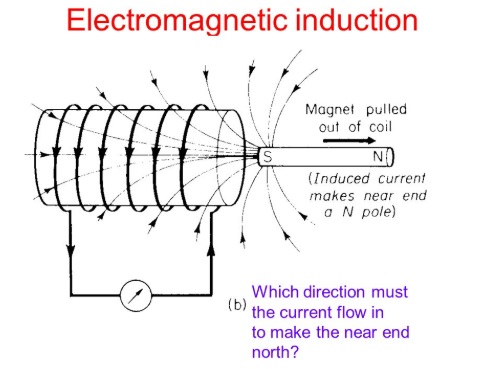# INTRODUCTION TO ELECTROMAGNETIC INDUCTION## Concept of Electromagnetic induction :

The phenomenon of electromagnetic Induction was discovered by oersted in 1820. Later faraday tried and succeeded in converting magnetism into electricity. All the electrical machines like generators, motors, Transformers
etc work on faradays laws of Electromagnetic Induction. Therefore it is necessary to have the knowledge of electro magnetic Induction.

### Faraday’s Laws of electromagnetic Induction :

First Law :

It states that whenever the magnetic flux linked with a circuit changes an emf is always induced in it or whenever a conductor is made to rotate in a magnetic field, hence cuts the magnetic flux and an emf is induced in the conductor.

Second law :

It states that the magnitude of the induced emf is equal to the rate of change of flux flux linkages i.e. It depends on number of turns of the coil and rate of change of flux.

Let N = No. of turns of coil
φ 1 = Initial value of flux through the coil ( wb )
φ 2 = Find value of flux through coil ( in wb )
t = Time in seconds during which the flux changed from φ 1 toφ 2
Flux linkage means the product of flux and the number of turns associated with it.
Initial flux likages = Nφ 1### Flemings right hand rule:

Flemings Right hand rule can be used to find out the direction of induced emf in a conductor cutting magnetic flux.
Hold the thumb and the first two fingers of the right hand mutually at right angles. Place the fore finger in the direction of the flux and turn the hand so that the thumb points in the direction of motion. The second finger will point in the direction of the induced emf.The direction of the induced emf is such that it tends to oppose the change in flux which induces it

Magnitude of induced emf : e = Blv Volts

B = Uniform magnetic field in webers
L = Length of conductor in mts.
V = Velocity of the conductor in mts / sec

Direction of induced emf :

Direction of induced emf depends upon fleming Right hand rule :
Force on a current carrying conductor is

F = BIL Newtons.
∴ B = Field Intensity in wb / m2
I = Current in Amps
L = Length of conduct in mts.
Field intensity inside a solenoid.### Types of E.M.F’s Dynamically and statically Induced E.M.F’s :

The emf produced by electromagnetic induction can be divided into two types.

1) Dynamically induced emf ( motionally)
2) Statically induced emf ( No motion )

The magnetic flux through a circuit or coil or conductor may be changed by various means, and by that change of flux linkages causing the production of induced emf.